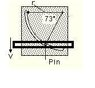# Curvilinear n&t motion question engineering mechanics

• Kasthuri
In summary, the pin is constrained to move in a circular slot of radius 39mm while being constrained to move down with a constant velocity of 8mm/s by a slotted bar. The magnitude of the acceleration of the pin at a specific position can be found by deriving the position function and plugging in the given time value. However, in the given attempt, the position of the pin was calculated incorrectly, resulting in a wrong answer for the acceleration. The correct position is x = 11.4mm and the correct acceleration is 3.7659 mm/s^2.

## Homework Statement

A pin is constrained to move in a circular slot of radius 39mm. At the same time a slotted bar also constrains the pin to move down with constant velocity 8mm/s. (as shown in attached diagram).

What is the magnitude of the acceleration of the pin for the position shown?

## Homework Equations

NB: instead of using n&t as hinted as suggested, I used x and y coordinate system

## The Attempt at a Solution

time taken for pin to move to position:

[39mm x sin(73)] / 8 mm/s = 4.6619... seconds

position of pin is:

x = 39 - sqrt(39^2 - 8^2*t^2)
so
x = 39 - sqrt(1521 - 64t^2)
y = -8t

pin's velocity is downward hence, it's acceleration is due to sideways movement: (x direction):

x^2 - 2*39*x = -64t^2 **

taking differential of **:
2*x*dx/dt - 2*39*dx/dt = -128t ***

taking differential of ***:
2*dx/dt + 2x*d^2x/dt^2 - 2*39*d^2x/dt^2 = -128 ****

Now, substitute t=4.6613.. into ** to get x = 17.83... mm

then substitute x and t values into *** to get dx/dt = 14.0959... mm/s

then substitute dx/dt into **** to get d^2x/dt^2 = 2.05515...mm/s^2

therefore,

However, my answer is wrong and I have no idea why?

Last edited:
Hi kasthuri, I don't know if I could help you but you apparently forgot to actually attach the "attached diagram" :)
side question: are you familiar with the lagrangian formalism ?

Sorry and thanks for telling me! I've attached it on my reply
Also, I don't know about the lagrangian formalism.
:)

#### Attachments

•diagram.JPG
5.5 KB · Views: 645
Hi, Katsthuri, sorry for my silly question about the lagrangian, I thought you were resolving a different kind of problem and I didn't read your whole post to well since the picture wasn't available